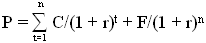# Yield to Call (YTC)

Updated November 23, 2020

## What is Yield to Call (YTC)?

Yield to call is a measure of the yield of a bond if you were to hold it until the call date.

## How Does Yield to Call (YTC) Work?

To understand yield to call, one must first understand that the price of a bond is equal to the present value of its future cash flows, as calculated by the following formula:where:

P = price of the bond
n = number of periods
C = coupon payment
r = required rate of return on this investment
F = principal at maturity
t = time period when payment is to be received

To calculate the yield to call, the investor then uses a financial calculator or software to find out what percentage rate (r) will make the present value of the bond's cash flows equal to today's selling price.

The big distinction with yield to call, however, is that the investor assumes that the bond is called at the earliest possible date rather than held to maturity. (To run the calculations assuming the bond is held to maturity would be to calculate the yield to maturity).

For example, say you own a Company XYZ bond with a \$1,000 par value and a 5% zero-coupon bonds that matures in three years. Also suppose this bond is callable in two years at 105% of par. To calculate the yield to call, you simply  pretend that the bond matures in two years rather than three, and calculate the yield accordingly. You should also consider the call price (105% of \$1,000, or \$1,050) as the principal at maturity (F). Thus, if this Company XYZ bond is selling for \$980 today, using the formula above we can calculate that the yield to call is 4.23%.

[Use our Yield to Call (YTC) Calculator to measure your annual return if you hold a particular bond until its first call date.]

## Why Does Yield to Call (YTC) Matter?

Although the yield to call calculation considers the three sources of potential return from a bond (coupon payments, capital gains, and reinvestment returns), some analysts consider it inappropriate to assume that the investor can reinvest the coupon payments at a rate equal to the yield to call. The yield to call makes two other tenuous assumptions: it assumes the investor will hold the bond until it is called, and it assumes the issuer will call the bond on one of the exact dates used in the analysis.

The true yield of a callable bond at any given price is usually lower than its yield to maturity because the call provisions limit the bond's potential price appreciation -- when interest rates fall, the price of a callable bond will not go any higher than its call price. This is because the issuer should act in the best interests of the company and call the bond as soon as it is favorable to do so.

As a result, investors usually consider the lower of the yield to call and the yield to maturity as the more realistic indication of the return an investor will actually receive on a callable bond. Some investors go a step further and calculate the yield to call not just for the first call date, but for all possible call dates. Then the investor compares all the calculated yields to call and yields to maturity and relies on the lowest of them, called the yield to worst.

All of our content is verified for accuracy by Paul Tracy and our team of certified financial experts. We pride ourselves on quality, research, and transparency, and we value your feedback. Below you'll find answers to some of the most common reader questions about Yield to Call (YTC).
##### Be the first to ask a question

Paul Tracy - 140Paul has been a respected figure in the financial markets for more than two decades. Prior to starting InvestingAnswers, Paul founded and managed one of the most influential investment research firms in America, with more than 2 million monthly readers.